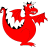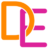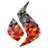22-26 March 2021

# Piola-mapped finite elements in Firedrake for linear elasticity and Stokes flow

Francis Aznaran (University of Oxford, 🇬🇧)
Patrick Farrell (University of Oxford, 🇬🇧)
Robert Kirby (Baylor University, 🇺🇸)
Tuesday session 2 (Zoom) (15:00–16:30 GMT)
View slides (pdf) (available under a CC BY 4.0 license)
10.6084/m9.figshare.14495295

Many finite element spaces are not preserved by the standard pullback to the reference cell. Robust implementation therefore requires studying the relation between degrees of freedom under pushforward, in order to obtain the correct bases on a generic physical triangle . In this work, we extend this transformation theory to vector- and tensor-valued elements mapped by the contravariant Piola transform. We apply this theory, and describe its efficient implementation inFiredrake, for the theMardal–Tai–Winther elements discretizing $$H(\mathrm{div})$$ for Stokes–Darcy flow, and theArnold–Winther elements discretizing $$H(\operatorname{div};\mathbb{S})$$ for the stress-displacement formulation of linear elasticity.

In particular, the Arnold–Winther elements were the first to stably enforce exact symmetry of the Cauchy stress tensor; we demonstrate how they may be efficiently mapped, while the few prior implementations are either custom-made for specific numerical experiments, or require the explicit element-by-element construction of the basis. Our novel implementation of these exotic elements composes inexpensively and automatically with the rest of the Firedrake code stack; numerical results are presented.

## References

•  R. C. Kirby. A general approach to transforming finite elements, The SMAI journal of computational mathematics 4, 197–224, 2018. [DOI: 10.5802/smai-jcm.33]